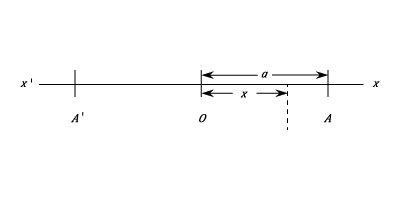I have forgotten

•http://facebook.com/
•https://www.google.com/accounts/o8/id
•https://me.yahoo.com

# Simple Harmonic Motion

An analysis of Simple Harmonic Motion.View other versions (3)

## Simple Harmonic Motion.

If a particle moves in a straight line in such a way that its acceleration is always directed towards a fixed point on the line and is proportional to the distance from the point, the particle is said to be moving in Simple Harmonic Motion.Let O be a fixed point on the line X'X and x the distance of the particle from O at any time t. Also, let the acceleration of the particle along OX as is a positive constant. No matter whether x is positive or negative the acceleration will be directed towards O.

If v is the velocity at time t, the acceleration will be in the direction OX and will be given by the differential relationship :-

This can be expressed as:

or,

In the initial stage of the motion v is negative as the particle is moving towards O

and,

When t = 0 x = a and = 0 and hence K = 0

and,

When Error: Invalid Equation cos nt = 0, and thus a particle starting from A moving towards O arrives in a time with a velocity of . It will continue along the straight line and its velocity will be zero when and . It will then return to O arriving when with a velocity and reach A in a time with zero velocity. The motion is then repeated indefinitely unless destroyed by some force.

Note:
• The time is called the Period of the oscillation and is the time for one complete cycle.
• If the frequency is f and the period , then .

Also,
• If the period of the motion is known, the motion is completely determined.
• The Period maybe written down at once if the magnitude of the acceleration for some value of x is known.
• The amplitude is determined by the initial displacement.

## Other Initial Conditions

If the the motion is started by giving the particle a velocity when its distance from O is , the type of motion is unchanged and the time is measured from this instant, instead of the instant when x = a. In this case the value of x at any instant is given by :-

where is a constant]

Now,

Also when

Then, And,

The Constant is called the Epoch of the motion. The Phase of the motion at time t is the time which has elapsed since the particle was at the positive end of its path. Thus the phase is less a multiple of the period.

Also,

In particular if , i.e. the particle starts from O and the amplitude is

Since the acceleration at any instant is

This is characteristic of Simple Harmonic Motion and its solution is given by:-

may be written down if the amplitude and the epoch are known.

## The Relation To Uniform Motion In A Circle.

If a particle is describing a circle of radius with uniform angular velocity , its orthogonal projection on a diameter of the circle moves on the diameter in simple harmonic motion of amplitude and period .

Let be the angle which the radius initially makes with the diameter X'OX . Then after a time t the angle made by the radius to the particle is . Hence, if P is the position of the particle at time t and N the foot of the perpendicular from P on OX, then:-

and the point moves with Simple Harmonic Motion of amplitude and period

Example:
[imperial]
##### Example - Simple Harmonic Motion
Problem
A particle moves with Simple Harmonic Motion in a straight line. Find the time of a complete oscillation if the acceleration is 4 ft/sec2, when the distance from the centre of the oscillation is 2 ft. If the Velocity with which the particle passes through the centre of oscillations is 8 ft./sec. find the amplitude.
Workings
If the acceleration is at a distance x from the centre then:

Hence the period is:

If the phase is zero when

where a is the amplitude. Then

And the value of v at the centre of oscillation is
and
Solution
The period is

and amplitude